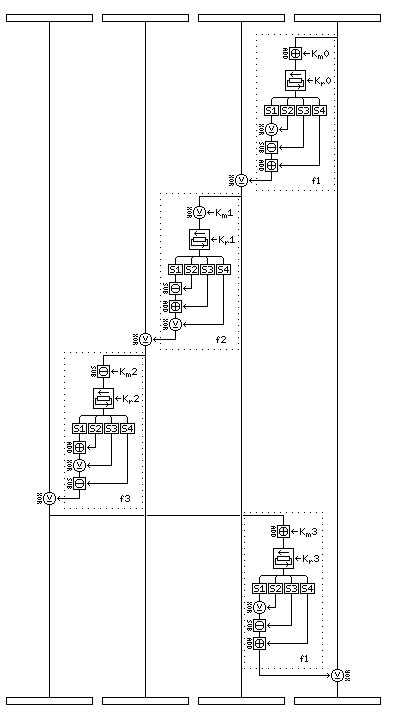[Next] [Up] [Previous] [Index]

# CAST-256

CAST-256 is the Canadian entry in the competition for the new Advanced Encryption Standard. It takes its name from the original CAST cipher, designed by Carlisle Adams and Stafford Tavares; they and others worked on the design of CAST-256. The original paper describing the cipher is at this location:

http://www.entrust.com/resources/pdf/cast-256.pdf

Unless it is chosen as the AES, licensing is likely to be required to use this submission as well as some others. (I am not certain of the actual status of this algorithm in that respect, however, and several algorithms originally only available with licensing have since been released.)

 I have been informed, by means of a communication from one Benjamin Choi, that the description of CAST-256 that I have given here contains errors, and I am inserting the corrections in this format for the time being until I can verify them myself.

It uses an f-function that produces a 32-bit output from a 32-bit input, and each round consists of modifying one 32-bit quarter of the block by XORing it with the f-function of another 32-bit quarter of the block. There are 48 rounds in CAST-256.

The rounds are organized in groups of four, called quadrounds. CAST-256 begins with six forwards quadrounds, and then continues with six reversed quadrounds, which are reversed exactly as would be required for decipherment. Thus, to perform CAST-256 decipherment, it is only necessary to change the order in which the subkeys are used.

Each round uses two subkeys, a "mashing" subkey 32 bits in length, and a rotation subkey 5 bits in length. A reverse quadround is reversed to the extent that the four rounds within it actually use their subkeys in reverse order; thus, the rounds of CAST-256 use their subkeys in this order:

```0 1 2 3 ... 22 23
27 26 25 24 31 30 29 28 35 34 33 32
39 38 37 36 43 42 41 40 47 46 45 44
```

The f-function in CAST-256 comes in three different flavors, with the positions in which the operations of addition, XOR, and subtraction are used changed. In the first type of f-function, the input first has the current mashing key added to it. Then, the result is given a circular left shift the extent of which is given by the current rotation key. Then, each of the four bytes of the result are used to select entries from one of the four S-boxes. The entries in the S-boxes are 32 bits wide. The entry in S1, selected by the first byte, first has the S2 entry selected by the second byte XORed to it, then the S3 entry selected by the third byte subtracted from it, then the S4 entry selected by the fourth byte added to it.

In the f2 function, the mashing key is XORed, the S2 entry is subtracted, the S3 entry is added, and the S4 entry is XORed.

In the f3 function, the mashing key is subtracted, the S2 entry is added, the S3 entry is XORed, and the S4 entry is subtracted.

 Instead of subtracting the mashing key from the input to the f3 function, that input should be subtracted from the mashing key to produce the result. (Possibly the other subtractions need to be reversed as well, or this may be the only subtraction to which this applies.)

The following diagram illustrates the operation of a forwards quadround:The block is divided into four 32-bit segments. First, an f1 f-function of the fourth segment is XORed to the third segment, then an f2 f-function of the third segment is XORed to the second segment, then an f3 f-function of the second segment is XORed to the first segment, and finally an f1 f-function of the first segment is XORed to the fourth segment.

### The Key Schedule

An operation called an "octave" is used to generate the CAST-256 key schedule. An octave is just like a forwards quadround, except it operates on a 256-bit block, and comprises eight rounds; first, an f1 f-function of the eighth segment is XORed to the seventh segment, and so on until an f2 f-function of the first segment is XORed to the eighth segment at the end.

CAST-256 may have a key that is 128, 160, 192, 224 or 256 bits long, providing two additional key lengths besides those required for an AES candidate. The 256-bit input to the first octave consists of the key padded with zero 32-bit words in the rightwards, later positions.

The subkeys used for the octaves are as follows: the first mashing subkey is 5A827999 in hexadecimal, and the first rotation subkey is 19 in hexadecimal.

Subsequent subkeys are formed by adding 6ED9EBA1 to the previous round's mashing subkey, and adding 17 (again, hexadecimal) to the previous round's rotation subkey. Needless to say, the two additions are modulo 2^32 and 2^5, respectively.

 In fact, the rotation subkeys 19 and 17 are actually decimal values. Also, two octaves are performed at a time before the 256-bit current value is added to the subkey material to be used.

The value of the block after each octave supplies enough subkey material for one quadround of the cipher. The eight 32-bit segments of the 256-bit block supply KR0, KM3, KR1, KM2, KR2, KM1, KR3, and KM0 in order: that is, the first segment supplies the first rotation key for the quadround (all but the last five bits are ignored), the second segment supplies the last mashing key for the quadround, and one proceeds onwards, forwards through the rotation keys and backwards through the mashing keys.

Since CAST-256 has 48 rounds in 12 quadrounds, twelve octaves are required to produce all the subkey material needed.

 The number of required octaves is doubled to twenty-four, based on the note above.

[Next] [Up] [Previous] [Index]